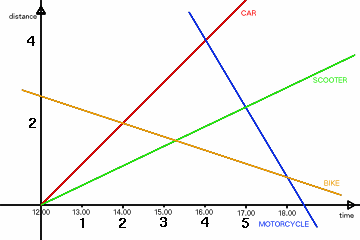#### You may also like### Pentagonal

Can you prove that the sum of the distances of any point inside a square from its sides is always equal (half the perimeter)? Can you prove it to be true for a rectangle or a hexagon?### A Scale for the Solar System

The Earth is further from the Sun than Venus, but how much further? Twice as far? Ten times?### Swimmers

Swimmers in opposite directions cross at 20m and at 30m from each end of a swimming pool. How long is the pool ?

# On the Road

##### Age 14 to 16Challenge Level

This solution was sent in by Ben at Madras College in St Andrews. Another good solution was sent in by Nicola at Madras College.

The car overtakes the scooter at 12.00. This point can be taken as the origin of a graph of distance against time.Assuming that the car travels at 1 unit per hour, the car therefore has equation y=x.

Now assume that the scooter travels at $a$ times the speed of the car, where $0 < a < 1$. So, it has equation $y=ax$.

Now for the motorcycle, whose line goes through $(4,4)$ and $(5,5a)$.
Gradient $= (5a-4)/(5-4) = 5a-4$
Substituting in the point $(5,5a)$,

$$\begin{eqnarray} y - b &=& m(x-a) \\ y - 5a &=& (5a-4)(x-5) \\ y &=& 5ax - 4x - 20a + 20 \end{eqnarray}$$

To find the intersection of the motorcycle and bike, we know that $x=6$.
Therefore $y-5a = (5a-4) \times 1$, and so $y = 10a-4$.

For the bike,

$$gradient = \frac{10a-4-2}{6-2} = \frac{10a-6}{4}$$

Using $(6, 10a-4)$, [Why not $(2,2)$? - Ed]

$$\begin{eqnarray} y -b &=& m(x-a) \\ y - (10a-4)& =& \frac{10a-6}{4}(x-6) \\ 4y - 40a + 16 &=& 10ax - 6x - 60a + 36 \\ 4y &=& 10ax - 6x - 20a + 20 \\ \end{eqnarray}$$

When the bike meets the scooter, $y=ax$, so $4y=4ax$.
Therefore

$$\begin{eqnarray} 10ax - 6x - 20a + 20 &=& 4ax \\ 6ax - 6x &=& 20a - 20 \\ 3ax - 3x &=& 10a - 10 \\ 3x(a-1) &=& 10(a-1) \\ 3x &=& 10 \\ x &=& 3\frac{1}{3} \end{eqnarray}$$

So, whatever the value of $a$, the scooter and bike meet 3 hours and 20 minutes after 12.00, at 15.20.